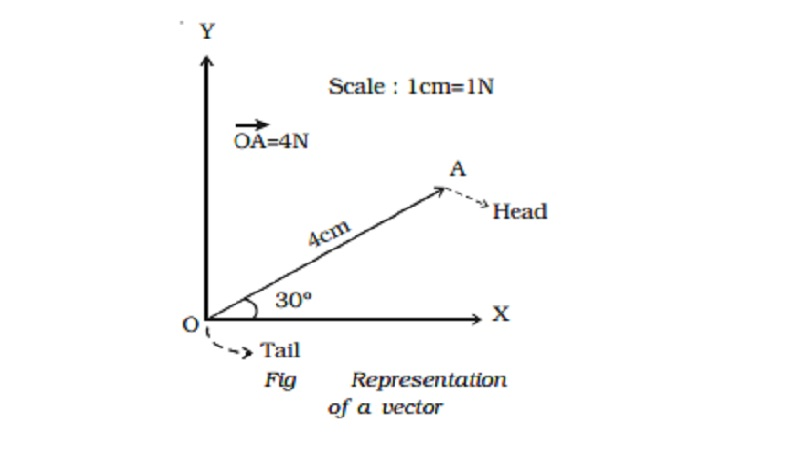Home | | Physics | | Physics | Representation of a vector quantities

# Representation of a vector quantitiesVector quantities are often represented by a scaled vector diagrams. Vector diagrams represent a vector by the use of an arrow drawn to scale in a specific direction. An example of a scaled vector diagram is shown in Fig

Scalar and vector quantities

A study of motion will involve the introduction of a variety of quantities, which are used to describe the physical world. Examples of such quantities are distance, displacement, speed, velocity, acceleration, mass, momentum, energy, work, power etc. All these quantities can be divided into two categories ? scalars and vectors.

The scalar quantities have magnitude only. It is denoted by a number and unit. Examples : length, mass, time, speed, work, energy,

temperature etc. Scalars of the same kind can be added, subtracted, multiplied or divided by ordinary laws.

The vector quantities have both magnitude and direction. Examples: displacement, velocity, acceleration, force, weight, momentum, etc.

Representation of a vector

Vector quantities are often represented by a scaled vector diagrams. Vector diagrams represent a vector by the use of an arrow drawn to scale in a specific direction. An example of a scaled vector diagram is shown in Fig .

From the figure, it is clear that1.  The scale is listed.

2.    A line with an arrow is drawn in a specified direction.

The magnitude and direction of the vector are clearly labelled. In the above case, the diagram shows that the magnitude is 4 N and direction is 30? to x-axis. The length of the line gives the magnitude and arrow head gives the direction. In notation, the vector is denoted in bold face letter such as A or with an arrow above the letter as Vector Vec(A), read as vector A or A vector while its magnitude is denoted by A or by||.

Study Material, Lecturing Notes, Assignment, Reference, Wiki description explanation, brief detail

Related Topics